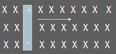# Motional EMF

• Motional emf is the voltage induced in a conductor moving through a magnetic field.• The magnetic field is into the page (-z) and the velocity is to the right; using the right hand rule, there is an upward force on the proton and a downward force on the electron pushing them to the extreme ends of the conductor as shown.

## Production of an Electric Field

• Since there is a separation of charge in the conductor, an electric field is produced in the conductor.
• Eventually, for the electron, the magnetic force (down) = the electric force (up) at equilibrium
• Therefore, qvB = qE; and E=vB
• Since V=Ed or in this case d=length of the conductor; V=El OR V=vBl OR V=Bl

## Derivation of Equation for ε For a Moving Conductor

• Since V=Ed or in this case d=length of the conductor; V=El OR V=vBL
• ε = Blv
• ε = induced emf, in Volts
• B = magnetic field, in T
• l = length of conducting bar, in m
• v = velocity of conductor, in m/s

## Potential Difference in the Bar

• Since the positive charges were forced to the top of the bar, it is at the higher potential
• Potential difference is maintained across a conductor as long as there is motion through the field.
• If the motion is reversed, the polarity of the potential difference is also reversed.

## A Moving Conductor as Part of a Closed Circuit

• Use the right hand rule for the current in the conductor to determine the direction of the magnetic force
• If the bar moves Δx in Δt, then …
• ΔΦ = BA = Bl(Δx) , if N=1 loop,
• ε = ΔΦ/Δt = (Bl Δx/Δt) = Blv
• The induced current, I = ε/R = Blv/R

(source)